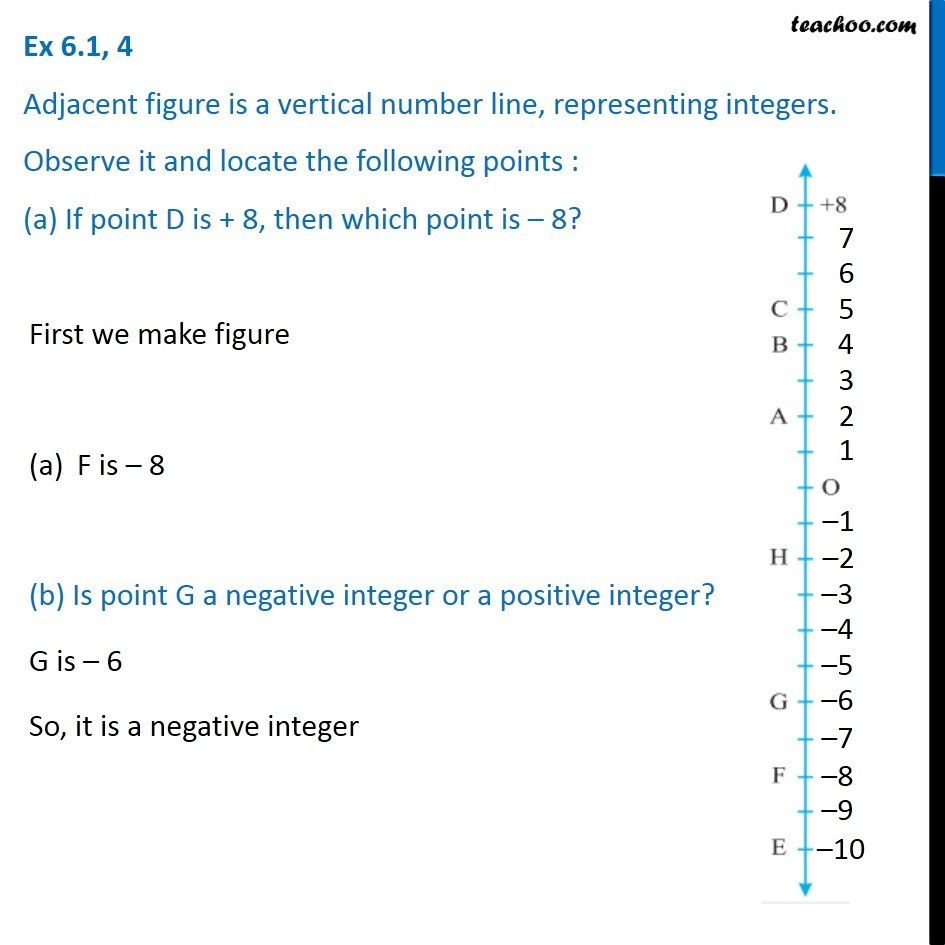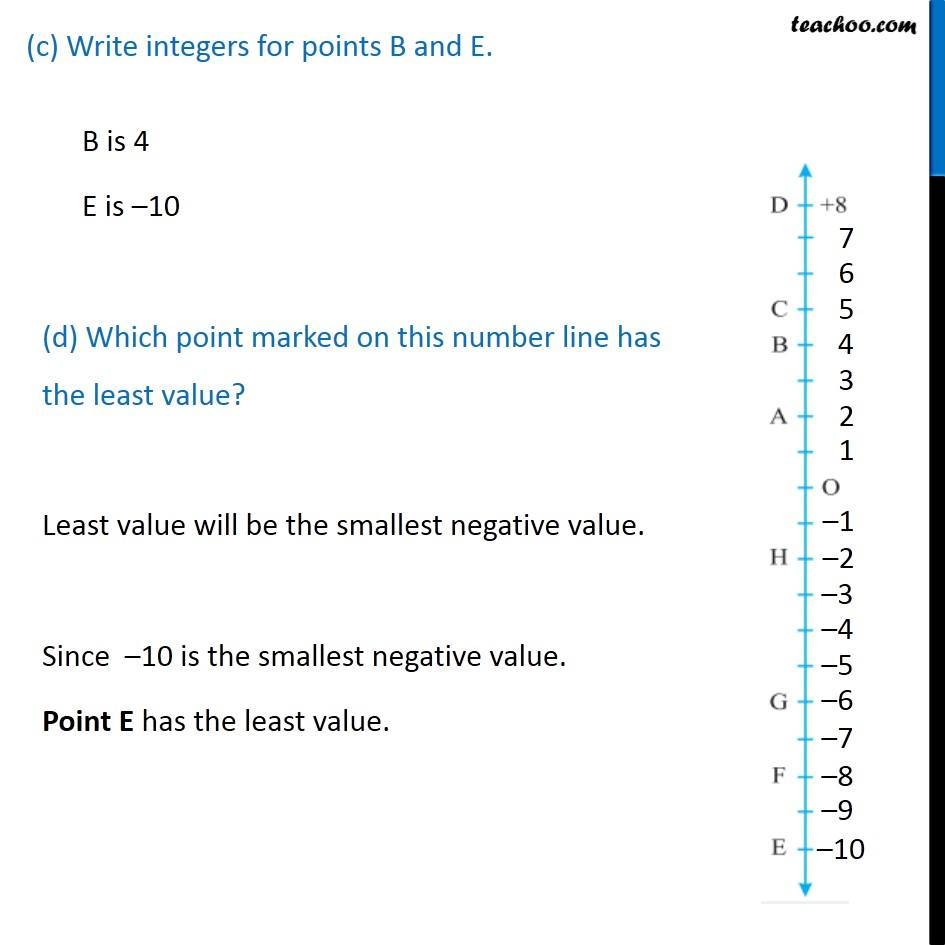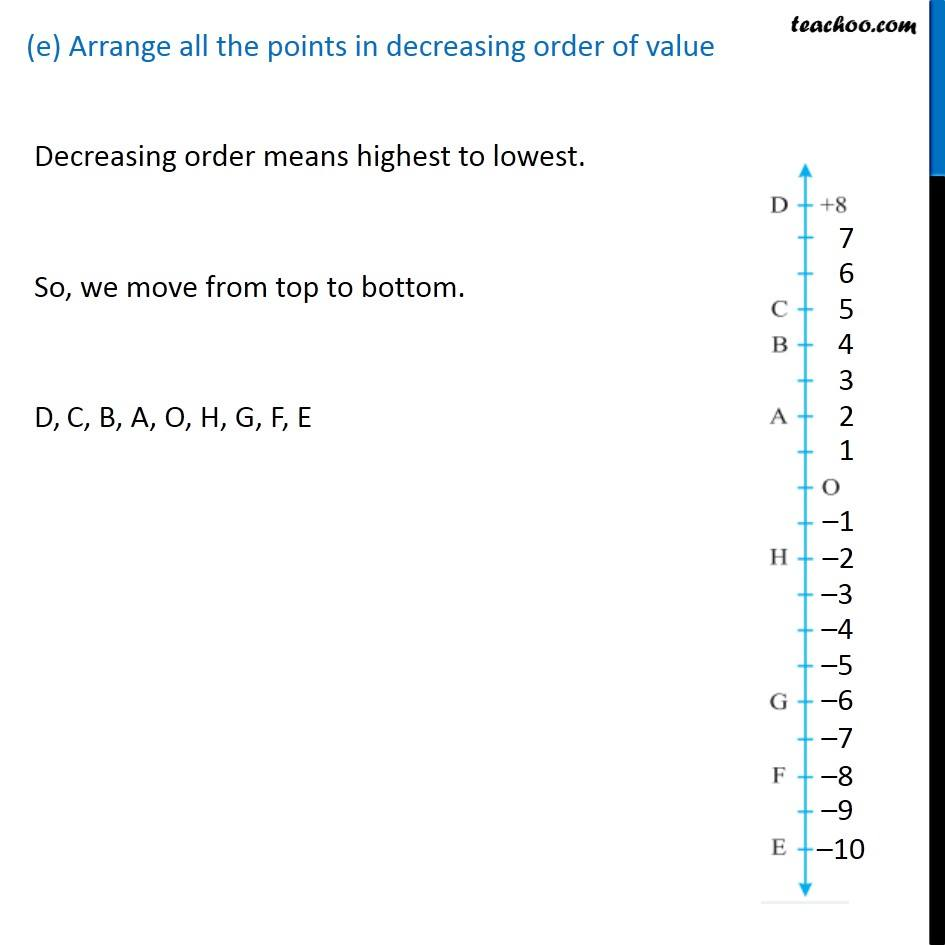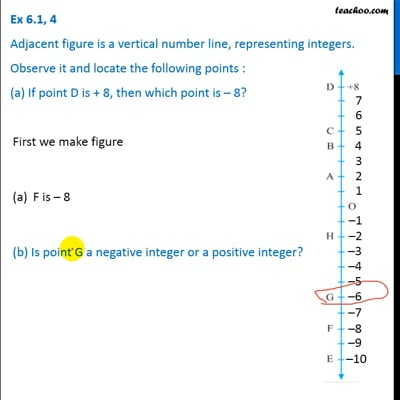Ex 6.1

Chapter 6 Class 6 Integers
Serial order wiseThis video is only available for Teachoo black users

### Transcript

Ex 6.1, 4 Adjacent figure is a vertical number line, representing integers. Observe it and locate the following points : (a) If point D is + 8, then which point is – 8? First we make figure F is – 8 (b) Is point G a negative integer or a positive integer? G is – 6 So, it is a negative integer (c) Write integers for points B and E. B is 4 E is –10 (d) Which point marked on this number line has the least value? Least value will be the smallest negative value. Since –10 is the smallest negative value. Point E has the least value. (e) Arrange all the points in decreasing order of valueDecreasing order means highest to lowest. So, we move from top to bottom. D,